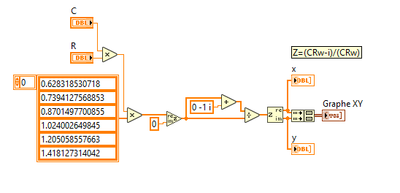LabVIEW

cancel
Showing results for
Did you mean:

Solved!
Go to solution

simulate complex impedance

Hi

I would like to plot complex impedance spectra (directly by using complex values), and I may not understand well or make a mistake I do not see.

For instance, for a RC, the impedance should be Z=(RCw-j)/(RCw)I do not obtain de half of the circle I expect...

thanks for help (I added the vi as well)

Regards

Message 1 of 5
(265 Views)

Re: simulate complex impedance

Hi

I do not know what the problem was, by writing differently, it works as it is supposed to :Message 2 of 5
(237 Views)

Re: simulate complex impedance

I do not know what the problem was,

You are now using a completely different formula!?

Message 3 of 5
(203 Views)

Re: simulate complex impedance

Hi

Yes, a different formula, but with complex function operations (still I don't know why the first case was not working as I expected).

N

Message 4 of 5
(195 Views)
Solution

Re: simulate complex impedance

the first formula was (CRw-i)/CRw, which resolves to 1-i/CRw. That is a line parallel to the imaginary (i) axis!

The 2nd formula describes the expected "circle" in the Re/Im plane…

Btw. you don't need to convert the complex data back to Re/Im arrays to create a plot for your XY graph: the graph can handle complex array data directly!

Message 5 of 5
(162 Views)